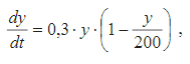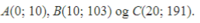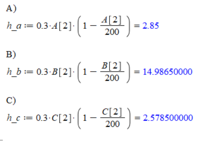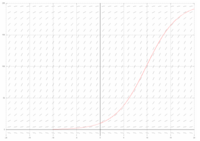# [Maple] - Drawing a curve through points with tangent slopes for each point.

#### emilo0212

##### New member
I wasn't sure how to title this, and it might seem like it's not related to differential equations, but it actually is and let me explain.

I've been given the following information:

The differential equation:Some points:The assignment:
a) Find the tangent slopes for the solutioncurve in the points A, B and C.
b) Draw the points A, B and C with each of their lineelements in a coordinatsystem.
c) Draw the solutioncurve through the points A, B and C.

I think it would be most relevant to give the results of the first question, as the second one is pretty straight forward. Here is my solution to a:I'm stuck at c right now and I'm not sure how these informations are related to each other and supposed to be used in a way that would draw a curve like the one below.

My friend gave me the solution to drawing it, but I have a hard time understand how he achieved it. Here is what the curve is supposed to look like:Would really appreciate information and/or examples with maple. Thx again!

#### Cubist

##### Junior Member
I suspect that the question is just asking for a sketch of the curve.

You have three points, which you can plot on an xy graph. Then you can draw small lines through each of the points using the associated gradients that you calculated. These lines will indicate the direction of the curve through the points. Then you can sketch a freehand curve through these points.

I am not familiar with "Maple".

It is possible to determine the exact equation of the curve. Is this what you want to do? Or maybe the Maple software enables you to plot a differential equation without solving it?

You seem to be aware of "differential equations". Are you on a mathematics course? If so then have you covered differential equations and the separation of variables yet?

I'm just trying to work out the best way to help you. This depends on your background.

#### Cubist

##### Junior Member
I just did a quick internet search "maple how to plot a de" and found the following

https://www.maplesoft.com/support/help/Maple/view.aspx?path=examples/deplot

https://www.maplesoft.com/support/help/Maple/view.aspx?path=DEtools/DEplot

--

If you want to see the exact solution of the differential equation click below for an "answer at the back of the book"...
This solution goes exactly through point A (and approximately goes through points B & C)...

$$\displaystyle t=\frac{10}{3}\ln\left(\frac{19y}{200-y}\right)$$

If you are good at integrations, and if you are interested, then I (or others) could show you how to obtain the above equation

You can see this ploted on GraphSketch (click)